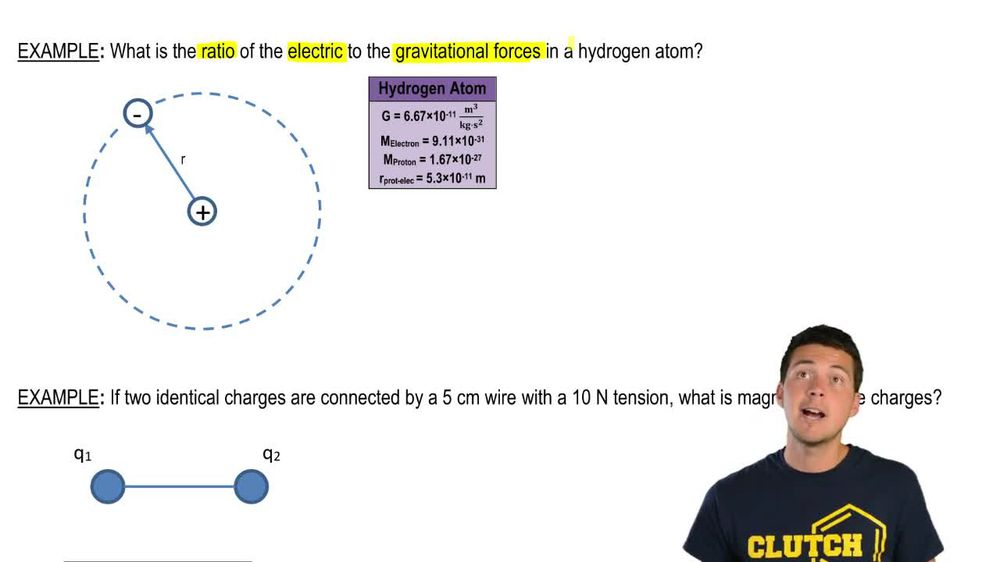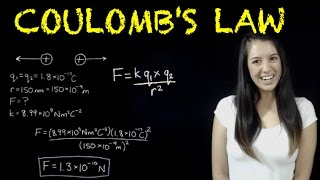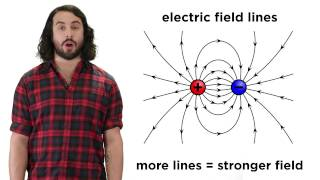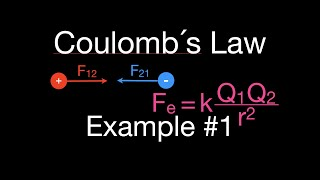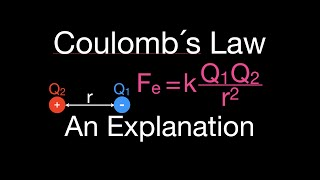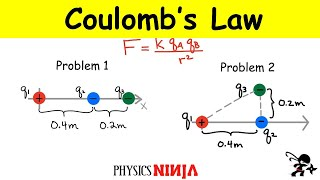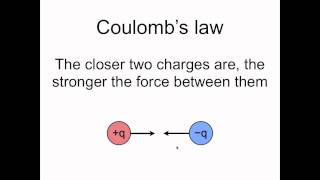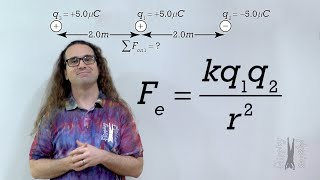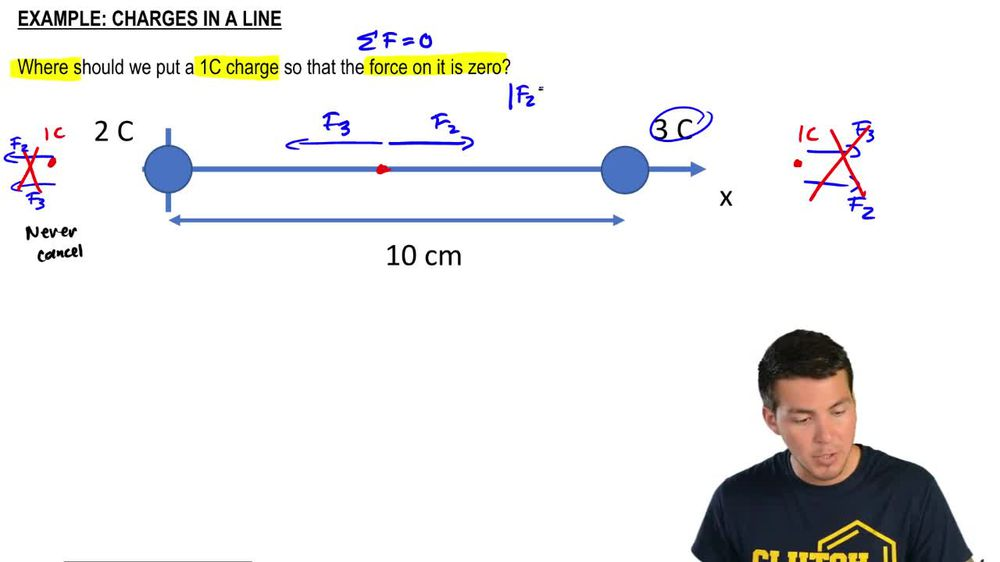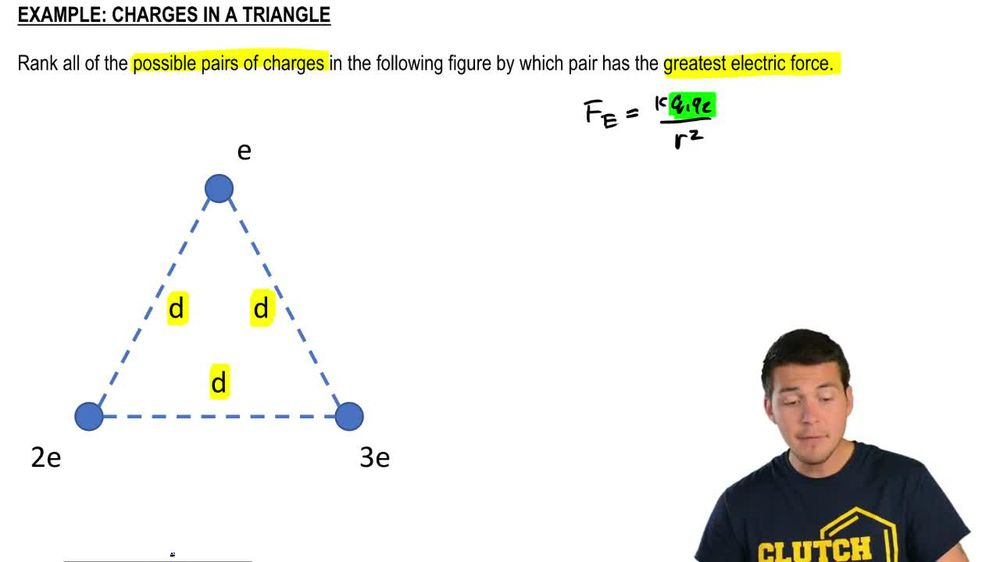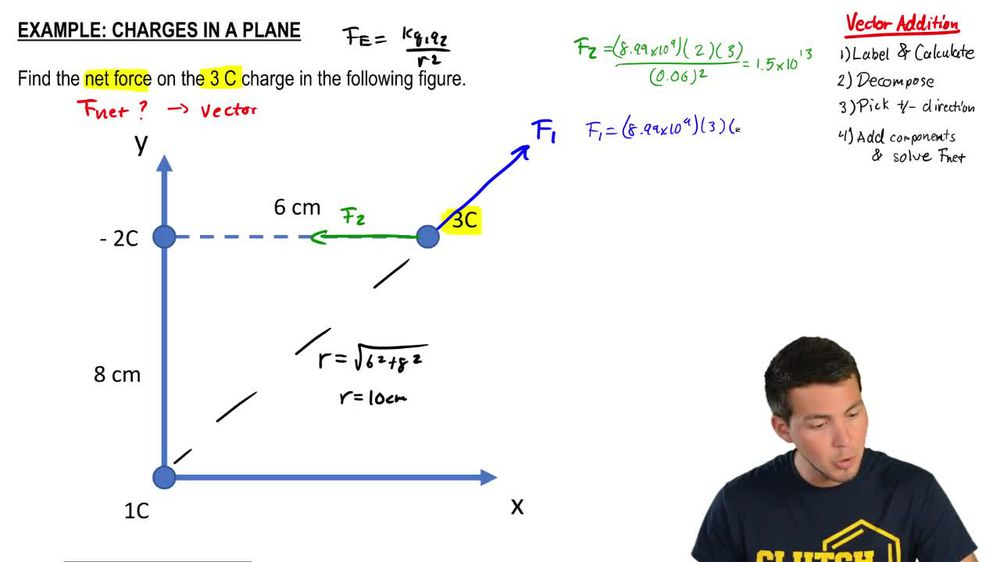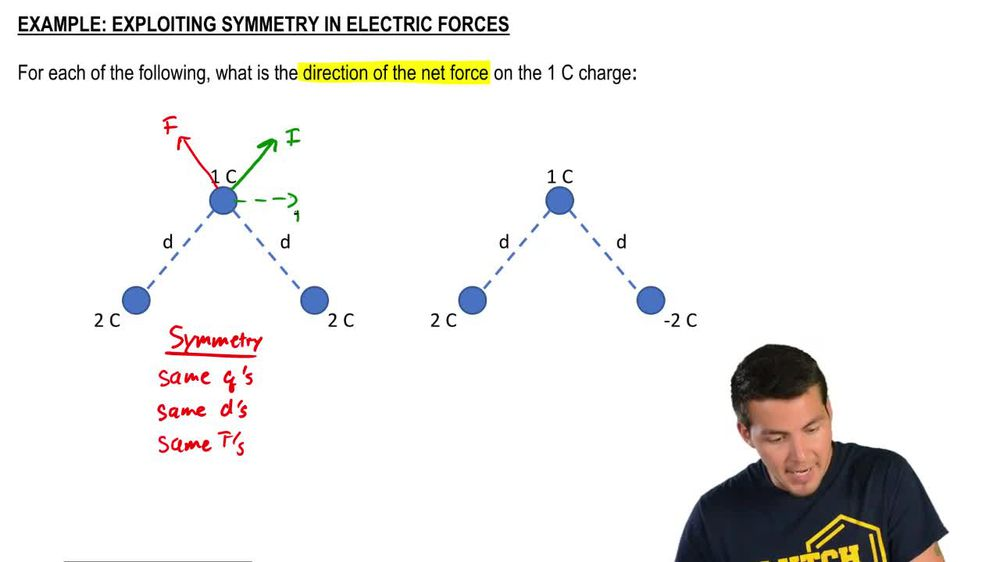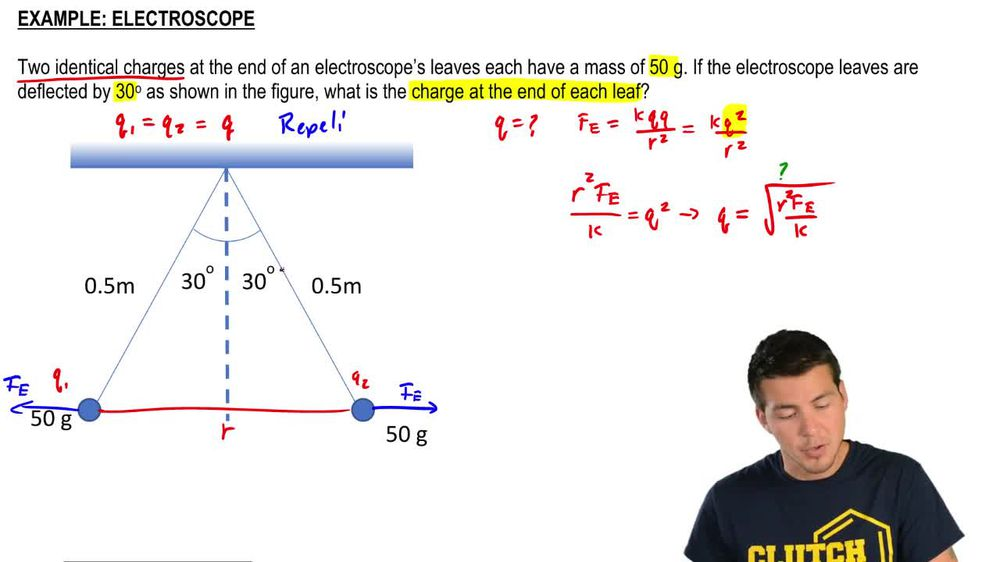Start typing, then use the up and down arrows to select an option from the list.
1. 24. Electric Force & Field; Gauss' Law2. Coulomb's Law (Electric Force)# Charges In A Triangle (Rank Force Pairs)

by Patrick Ford
136 views
3
3
Hey, guys. So in this problem, we have to rank although the possible pairs of charges in this diagram by which one has the greatest possible electric force. So we're talking about cool owns law and specifically for point charges. So we've got to use columns. Law K Q one Q two divided by the distance between them square. That's little are in this diagram, though we actually have the distance as D, but it's the same exact distance through all of the points in this triangle, which means that this D isn't gonna be a factor in determining which one is the strongest in which one is the weakest. Instead, what we have to dio is we have to just look at the product of the charges themselves, and we have three pairs that we're gonna discuss. We're going to discuss the pair between two and three e to e and sorry to an e two and three and then e and three. So I've got these little loops right here that represent those pears, and all we have to do is just compare the magnitude of the charges involved. Now, all these air like charges that all exert repulsive forces on each other, so we just have to figure what Q one Q two is Now. I've got to e and E and remember that e stands for the elementary charge, so I've got to eat times E. So, in other words, that's just gonna be two e squared. Remember that E is just 1.6 times 10 to the minus 19 just stands for, like, the electron or the country charge. And if I do the same exact thing over here, I've got Q one. Q two is equal to two e times three. And then that's just gonna equal six e squared. And then I've got Zoo Wannabe, the minus sign and then for the last one. I've got e times three. So that's just gonna be three e squared, right? That's Q one Q. Two. All right, so we're just gonna basically plug in these things if we're actually trying to figure out what the forces are inside for Q one. Q. Two, Which one is the greatest? Well, the six e squared is first, and then the three e squared is next. And then, finally, this two e squared is third, so that's That's the rank in terms off, sort of descending, greatest electric force. Alright, guys, let me know if you guys have any questions with that.